# Money in the Bank: Two-Digit Addition

This little piggy said "wee! wee! wee!" all the way to the bank...because he was loaded! Ask your second grader to do some mental maths to figure out how much moolah each pig is carrying.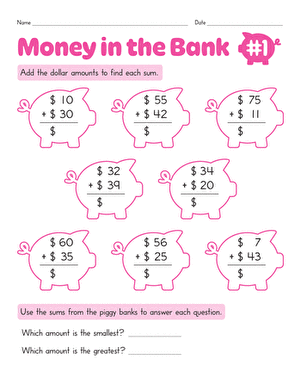### Money in the Bank: Two-Digit Addition #1

How much money is in each piggy bank? Give your second grader practise with double-digit addition as he gets more familiar with mental maths.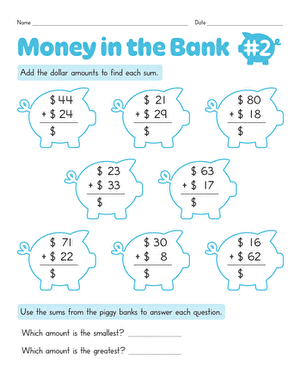### Money in the Bank: Two-Digit Addition #2

Use this colorful worksheet to boost your second grader's maths skills. She'll add up all the problems to see how much money is in each piggy bank.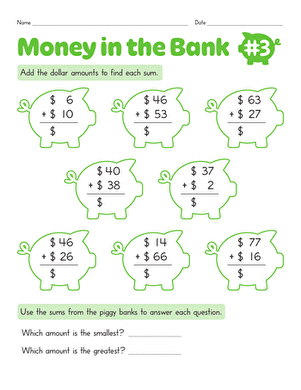### Money in the Bank: Two-Digit Addition #3

Don't break the bank! Can your second grader use double-digit addition to determine how much money is in each of these piggy banks?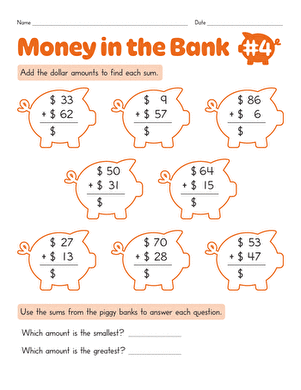### Money in the Bank: Two-Digit Addition #4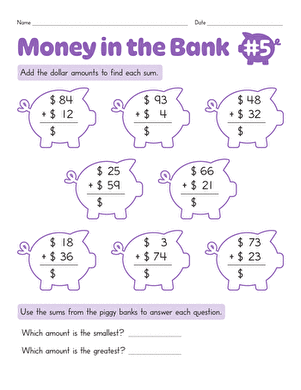### Money in the Bank: Two-Digit Addition #5

How much money does each little piggy have? Give your second grader's double-digit addition skills a work out with this fun printable.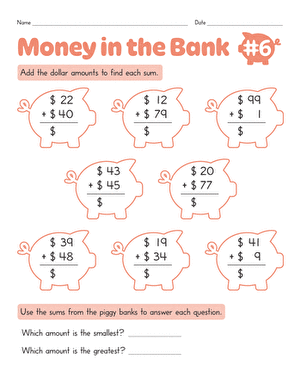### Money in the Bank: Two-Digit Addition #6

Can your second grader find out how much money is in each piggy bank? He'll use his addition skills to count up the dollars!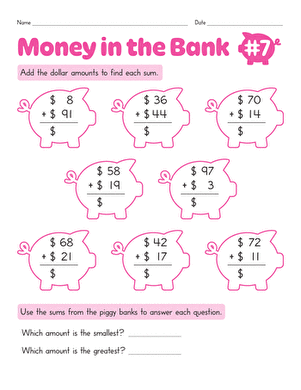### Money in the Bank: Two-Digit Addition #7

Which piggy bank has the most money? Your second grader will boost her double-digit addition and mental maths ability as she works to find out.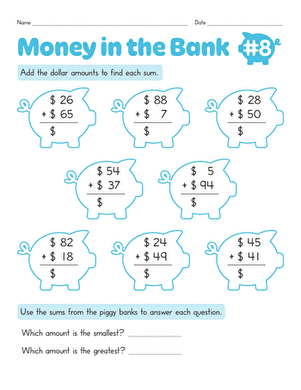### Money in the Bank: Two-Digit Addition #8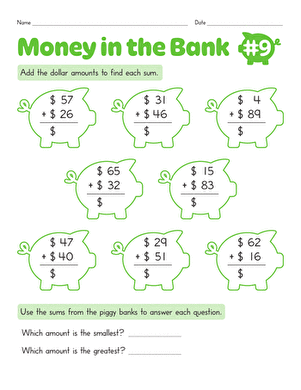### Money in the Bank: Two-Digit Addition #9

This charming piggy bank worksheet gives your second grader a chance to exercise his two-digit addition and mental maths skills.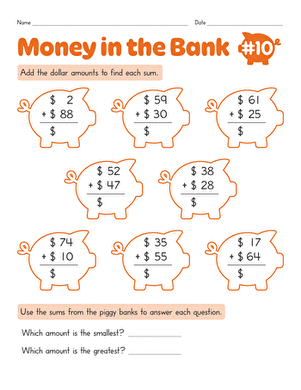### Money in the Bank: Two-Digit Addition #10

Your second grader will get a mental maths boost as he practices two-digit addition to figure out how much money is in each piggy bank.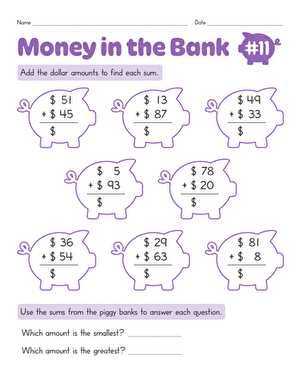### Money in the Bank: Two-Digit Addition #11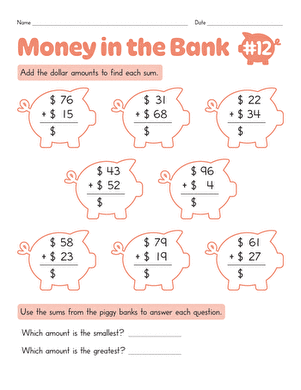### Money in the Bank: Two-Digit Addition #12

Watch your second grader boost his mental maths skills as he works through these addition problems to find out how much money each piggy bank holds.### Money in the Bank: Two-Digit Addition #13

In no time at all, you'll see your second grader boost her double-digit addition skills and practise mental maths with this piggy bank worksheet.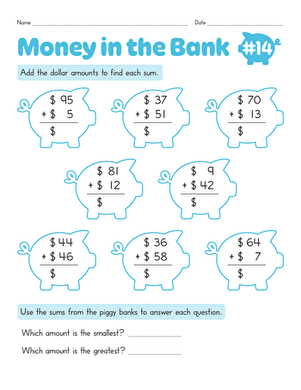### Money in the Bank: Two-Digit Addition #14

How many dollars are in each piggy bank? Watch your second grader use his addition skills to count up the dollars, which strengthens his mental maths, too.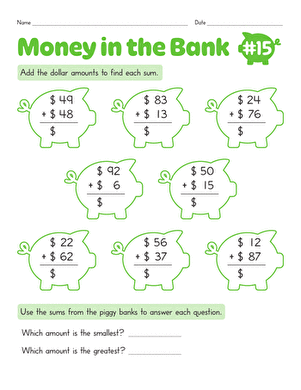### Money in the Bank: Two-Digit Addition #15

Let your second grader work through a heap of double-digit addition problems to find the piggy bank that holds the most and least amount of money.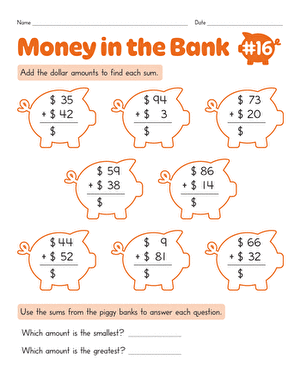### Money in the Bank: Two-Digit Addition #16

Kids will solve maths problems to find out how much money is in each piggy bank. Exercise mental maths skills with this two-digit addition worksheet.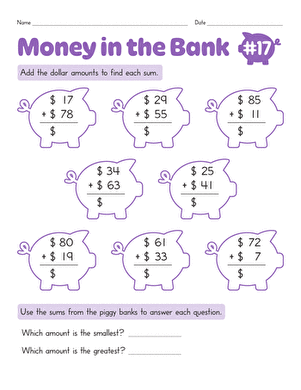### Money in the Bank: Two-Digit Addition #17

Find out how much money is in the bank with this two-digit addition piggy bank worksheets. Use mental maths to solve addition problems in this maths worksheet.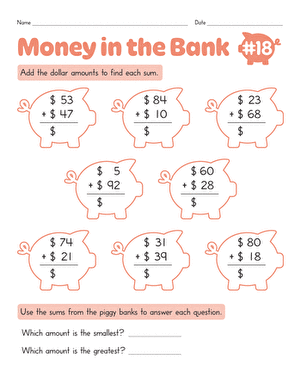### Money in the Bank: Two-Digit Addition #18

Use mental maths to work through two-digit addition problems in this maths worksheet. Kids will solve to find out how much money is in each piggy bank.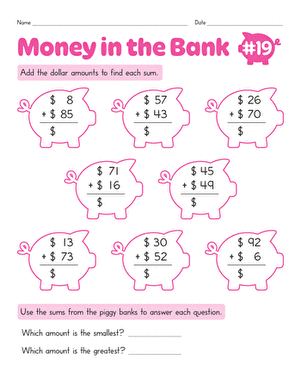### Money in the Bank: Two-Digit Addition #19

Use this piggy bank maths worksheet to practise two-digit addition. Try this maths worksheet with your child.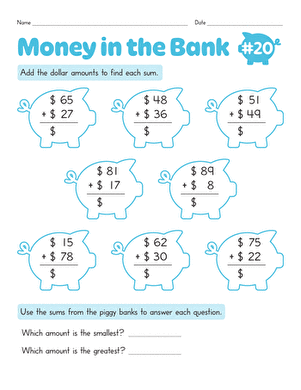### Money in the Bank: Two-Digit Addition #20

Work on two-digit addition with this piggy bank maths worksheet. Kids will solve problems to find out how much money is in each bank.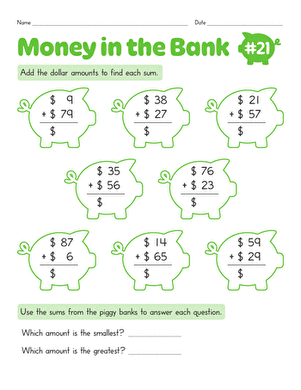### Money in the Bank: Two-Digit Addition #21

Solve two-digit addition problems with piggy bank maths. Find out how much money is in each piggy bank with this maths worksheet.### Money in the Bank: Two-Digit Addition #22

Use this colorful two-digit addition worksheet with your child. Solve this addition problems to find out how much money is in each piggy bank.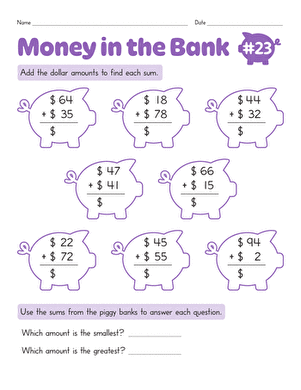### Money in the Bank: Two-Digit Addition #23

Try this piggy-bank-themed worksheet to practise addition with your child. Solve addition problems to find out how much money is in each piggy bank.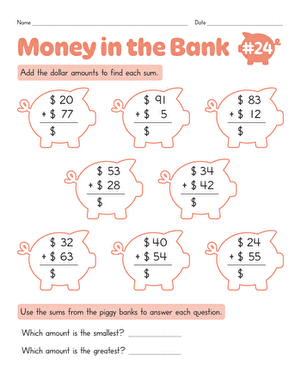### Money in the Bank: Two-Digit Addition #24

Kids will do two-digit addition problems to find out how much money is in each piggy bank in this worksheet. practise maths skills with this worksheet.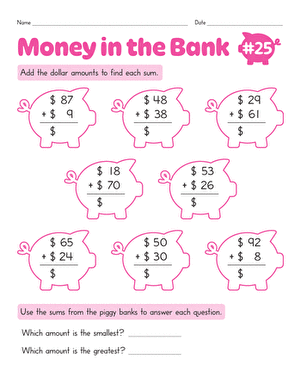### Money in the Bank: Two-Digit Addition #25

Solve two-digit addition problems in this piggy-bank-themed worksheet. Use your maths skills to figure out how much money is in each piggy bank.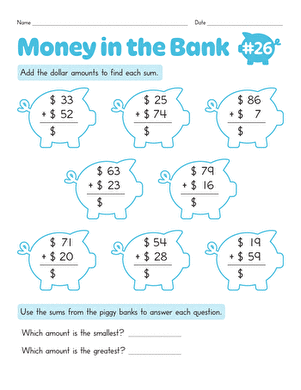### Money in the Bank: Two-Digit Addition #26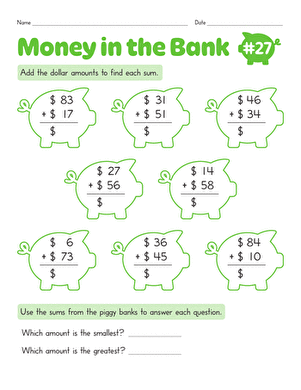### Money in the Bank: Two-Digit Addition #27

Kids will solve two-digit addition problems in this piggy-bank maths worksheet. Find out how much money is in each piggy bank through addition.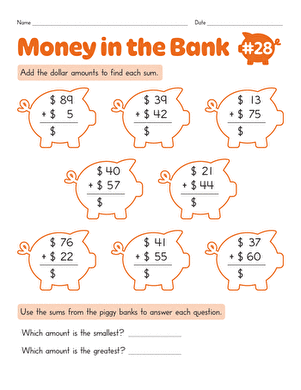### Money in the Bank: Two-Digit Addition #28

Use this piggy-bank maths worksheet with your young mathematician. Find out how much money is in each piggy with double-digit addition.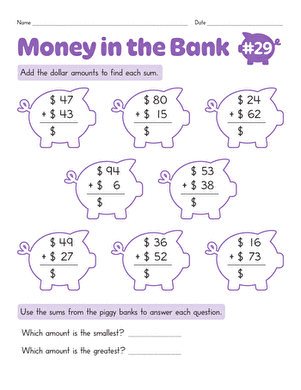### Money in the Bank: Two-Digit Addition #29

Try this piggy-bank-themed addition worksheet with your child. Your child will figure out how much money is in each piggy while practising adding double digits.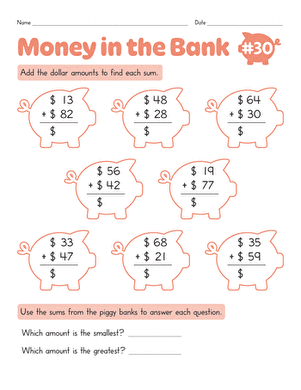### Money in the Bank: Two-Digit Addition #30

Create new collection

0

### New Collection>

0Items

What could we do to improve Education.com?